In this tutorial we will learn how to work with comma separated (CSV) files in Python and Pandas. We will get an overview of how to use Pandas to load CSV to dataframes and how to write dataframes to CSV.

In the first section, we will go through, with examples, how to read a CSV file, how to read specific columns from a CSV, how to read multiple CSV files and combine them to one dataframe, and, finally, how to convert data according to specific datatypes (e.g., using Pandas read_csv dtypes). In the last section we will continue by learning how to write CSV files. That is, we will learn how to export dataframes to CSV files.

## Pandas Import CSV from the Harddrive

In the first example of this Pandas read CSV tutorial we will just use read_csv to load CSV to dataframe that is in the same directory as the script. If we have the file in another directory we have to remember to add the full path to the file. Here’s the first, very simple, Pandas read_csv example:

The data can be downloaded here but in the following examples we are going to use Pandas read_csv to load data from a URL.

## Pandas Read CSV from a URL

In the next read_csv example we are going to read the same data from a URL. It’s very simple we just put the URL in as the first parameter in the read_csv method:

As can be seen in the image above we get a column named ‘Unamed: 0’. We can also see that it contains numbers. Thus, we can use this column as index column. In the next code example we are going to use Pandas read_csv and the index_col parameter.  This parameter can take an integer or a sequence. In our case we are going to use the integer 0 and we will get a way nicer dataframe: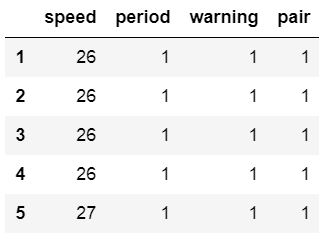The index_col parameter also can take a string as input and we will now use a different datafile. In the next example we will read a CSV into a Pandas dataframe and use the idNum column as index.Note, to get the above output we used Pandas iloc to select the first 7 rows. This was done to get an output that could be easier illustrated. That said, we are now continuing to the next section where we are going to read certain columns to a dataframe from a CSV file.

## Pandas Read CSV usecols

In some cases we don’t want to parse every column in the csv file. To only read certain columns we can use the parameter usecols. Note, if we want the first column to be index column and we want to parse the three first columns we need to have a list with 4 elements (compare my read_excel usecols example here):

Of course, using read_csv usecols make more sense if we had a CSV file with more columns.  We can use Pandas read_csv usecols with a list of strings, as well. In the next example we return to the larger file we used previously:

## Pandas Read CSV: Remove Unnamed Column

In some of the previous read_csv example we get an unnamed column. We have solved this by setting this column as index or used usecols to select specific columns from the CSV file. However, we may not want to do that for any reason. Here’s one example on how to use Pandas read_csv to get rid of the column “Unnamed:0”: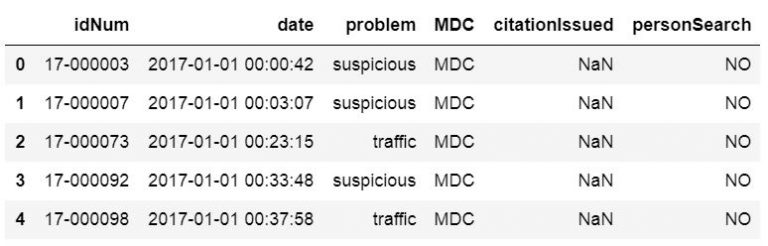It’s of course also possible to remove the unnamed columns after we have loaded the CSV to a dataframe. To remove the unnamed columns we can use two different methods; loc and drop, together with other Pandas dataframe methods. When using the drop method we can use the inplace parameter and get a dataframe without unnamed columns.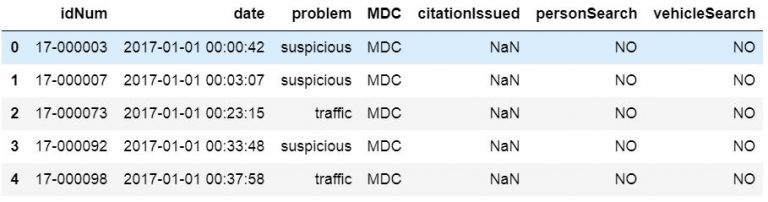To explain the code example above; we select the columns without columns that containing the string ‘unnamed’. Furthermore, we used the case parameter so that the contains method is not case-sensitive. Thus, we will get columns named “Unnamed” and “unnamed”. In the first row, using Pandas drop, we are also using the inplace parameter so that it changes our dataframe. The axis parameter, however, is used to drop columns instead of indices (i.e., rows).

## Read CSV and Missing Values

If we have missing data in our CSV file and it’s coded in a way that make it impossible for Pandas to find them we can use the parameter na_values. In the example below the amis.csv file have been changed and there are some cells with the string “Not Available”.

That is, we are going to change “Not Available” to something that we easily can remove when carrying out data analysis later.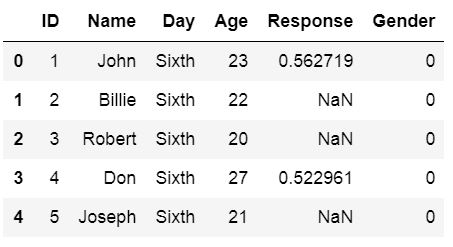## Reading CSV and Skipping Rows

What if our data file(s) contain information on the first x rows? For instance, how can we skip the first three rows in a file looking like this: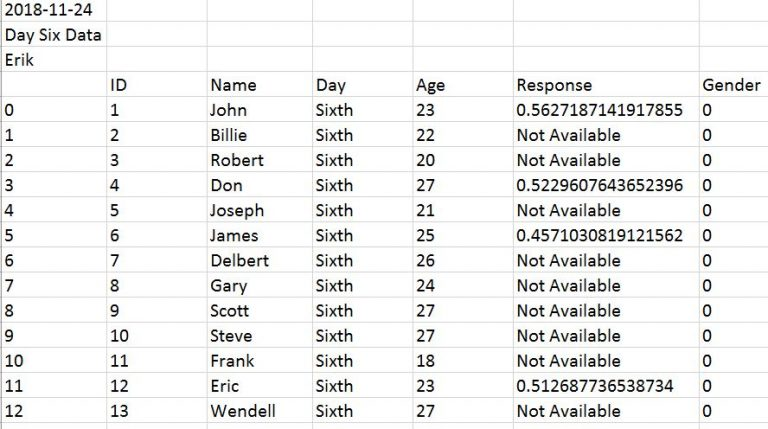We will now learn how to use Pandas read_csv and skip x amount of row. Luckily, it’s very simple we just use the skiprows parameter. In the following example we are using read_csv and skiprows=3 to skip the first 3 rows.

### Pandas read_csv skiprows example: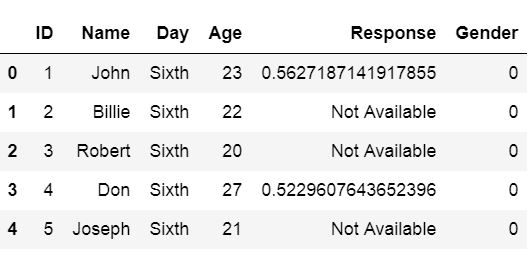Note we can obtain the same result as above using the header parameter (i.e., data = pd.read_csv(‘Simdata/skiprow.csv’, header=3)).

## How to Read Certain Rows using Pandas

If we don’t want to read every row in the CSV file we ca use the parameter nrows. In the next example below we read the first 8 rows of a CSV file.

##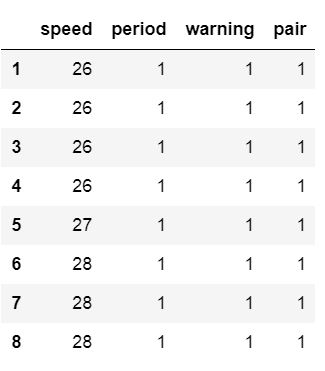If we want to select random rows we can load the complete CSV file and use Pandas sample to randomly select rows (learn more about this by reading the Pandas Sample tutorial).

## Pandas read_csv dtype

We can also set the data types for the columns. Although, in the amis dataset all columns contain integers we can set some of them to string data type. This is exactly what we will do in the next Pandas read_csv pandas example. We will use the Pandas read_csv dtype parameter and put in a dictionary: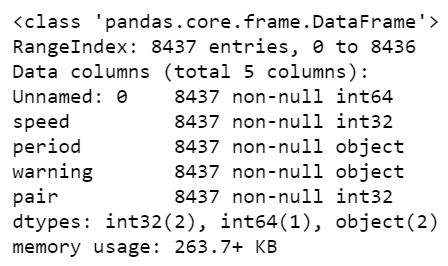It’s ,of course, possible to force other datatypes such as integer and float. All we have to do is change str to float, for instance (given that we have decimal numbers in that column, of course).

## Load Multiple Files to a Dataframe

If we have data from many sources such as experiment participants we may have them in multiple CSV files. If the data, from the different CSV files, are going to be analyzed together we may want to load them all into one dataframe.  In the next examples we are going to use Pandas read_csv to read multiple files.

First, we are going to use Python os and fnmatch to list all files with the word “Day” of the file type CSV in the directory “SimData”. Next, we are using Python list comprehension to load the CSV files into dataframes (stored in a list, see the type(dfs) output).

Finally, we use the method concat to concatenate the dataframes in our list. In the example files there is a column called ‘Day’ so that each day (i.e., CSV file) is unique.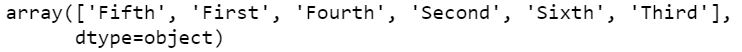The second method we are going to use is a bit simpler; using Python glob. If we compare the two methods (os + fnmatch vs. glob) we can see that in the list comprehension we don’t have to put the path. This is because glob will have the full path to our files. Handy!

If we don’t have a column, in each CSV file, identifying which dataset it is (e.g., data from different days) we could apply the filename in a new column of each dataframe:

## How to Write CSV files in Pandas

In this section we will learn how to export dataframes to CSV files. We will start by creating a dataframe with some variables but first we start by importing the modules Pandas:

The next step is to create a dataframe. We will create the dataframe using a dictionary. The keys will be the column names and the values will be lists containing our data: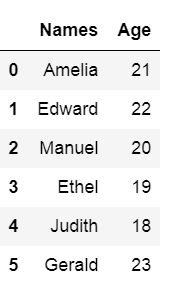Then we write the dataframe to CSV file using Pandas to_csv method. In the example below we don’t use any parameters but the path_or_buf which is, in our case, the file name.

Here’s how the exported dataframe look like: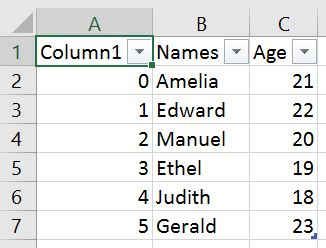As can be seen in the image above we get a new column when we are not using any parameters. This column is the index column from our Pandas dataframe. We can use the parameter index and set it to False to get rid of this column.

### How to Read Multiple Dataframes to one CSV file

If we have many dataframes and we want to export them all to the same CSV file it is, of course, possible. In the Pandas to_csv example below we have 3 dataframes. We are going to use Pandas concat with the parameters keys and names.

This is done to create two new columns, named Group and Row Num. The important part is Group which will identify the different dataframes. In the last row of the code example we use Pandas to_csv to write the dataframes to CSV.

In the CSV file we get 4 columns. The keys parameter with the list ([‘Group1’, ‘Group2’, ‘Group3’]) will enable identification of the different dataframes we wrote. We also get the column “Row Num” which will contain the row numbers for each dataframe: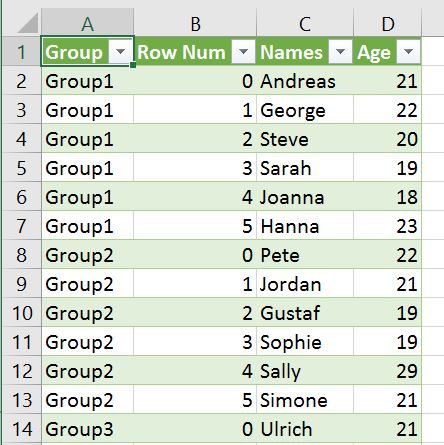## Conclusion

In this tutorial we have learned about importing CSV files into Pandas dataframe. More Specifically, we have learned how to:

• Load CSV files to dataframe
• locally
• from the WEB
• Read certain columns
• Remove unnamed columns
• Handle missing values
• Skipping rows and reading certain rows
• Changing datatypes using dtypes
• Reading many CSV files
• Saving dataframes to CSV

## Be First to Comment

This site uses Akismet to reduce spam. Learn how your comment data is processed.